# CBSE Class 9 Science Structure of the Atom assignment

Read and download free pdf of CBSE Class 9 Science Structure of the Atom assignment. Get printable school Assignments for Class 9 Science. Standard 9 students should practise questions and answers given here for Science in Grade 9 which will help them to strengthen their understanding of all important topics. Students should also download free pdf of Printable Worksheets for Class 9 Science prepared as per the latest books and syllabus issued by NCERT, CBSE, KVS and do problems daily to score better marks in tests and examinations

## Structure Of The Atom Class 9 Science Assignment Pdf

Class 9 Science students should refer to the following printable assignment in Pdf for Structure Of The Atom in standard 9. This test paper with questions and answers for Grade 9 Science will be very useful for exams and help you to score good marks

### Class 9 Science Assignment for Structure Of The Atom

MCQs

Question : Rutherford's alpha-particle scattering experiment was responsible for the discovery of
(a) Atomic nucleus
(b) Electron
(c) Proton
(d) Neutron
► (a) Atomic nucleus

Question : Isotopes of an element have
(a) the same physical properties
(b) different chemical properties
(c) different number of neutrons
(d) different atomic numbers
► (c) different number of neutrons

Question : Number of valence electrons in Cl -ion are:
(a) 16
(b) 8
(c) 17
(d) 18
► (b) 8

Question : Which one of the following is a correct electronic configuration of sodium?
(a) 2, 8
(b) 8, 2, 1
(c) 2, 1, 8
(d) 2, 8, 1
► (d) 2, 8, 1

Question : For the following statements, write T for 'True' and F for 'False'.

(a) J.J. Thomson proposed that the nucleus of an atom contains only nucleons.
► False

(b) A neutron is formed by an electron and a proton combining together.
Therefore, it is neutral.
► False

(c) The mass of an electron is about 1 / 2000times that of proton.
► True

(d) An isotope of iodine is used for making tincture iodine, which is used as a medicine.
► False

Question: Rutherford’s alpha – particle scattering experiment was responsible for the discovery of
(a) Atomic nucleus
(b) Electron
(c) Proton
(d) Neutron

Question: Isotopes of an element have
(a) The same physical properties
(b) Different chemical properties
(c) Different number of neutrons
(d) Different atomic numbers.

Question: Number of valence electrons in Cl- ion are:
(a) 16
(b) 8
(c) 17
(d) 18

Question: Which one of the following is a correct electronic configuration of Sodium?
(a) 2, 8
(b) 8, 2, 1
(c) 2, 1, 8
(d) 2, 8, 1

Question: Complete the following table.Question : What are canal rays?
Answer : Canal rays are positively charged radiations that can pass through perforated cathode plate. These rays consist of positively charged particles known as protons.

Question : If an atom contains one electron and one proton, will it carry any charge or not?
Answer : An electron is a negatively charged particle, whereas a proton is a positively charged particle. The magnitude of their charges is equal.
Therefore, an atom containing one electron and one proton will not carry any charge. Thus, it will be a neutral atom.

Question : On the basis of Thomson's model of an atom, explain how the atom is neutral as a whole.
Answer : As per Thomson’s model of the atom, an atom consists both negative and positive charges which are equal in number and magnitude. So, they balance each other as a result of which atom as a whole is electrically neutral.

Question : On the basis of Rutherford's model of an atom, which subatomic particle is present in the nucleus of an atom?
Answer :  On the basis of Rutherford's model of an atom, protons are present in the nucleus of an atom.

Question : Draw a sketch of Bohr's model of an atom with three shells.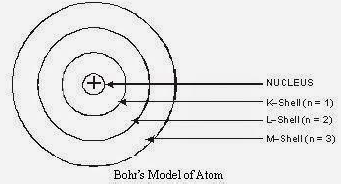Bohr's Model of an atom with three shells

Question : What do you think would be the observation if the α-particle scattering experiment is carried out using a foil of a metal other than gold?
Answer :  If α-particle scattering experiment is carried out using a foil of any metal as thin as gold foil used by Rutherford, there would be no change in observations. But since other metals are not so malleable so, such a thin foil is difficult to obtain. If we use a thick foil, then more α-particles would bounce back and no idea about the location of positive mass in the atom would be available with such a certainty.

Question : Name the three sub-atomic particles of an atom.
Answer :  The three sub-atomic particles of an atom are:
(i) Protons
(ii) Electrons, and
(iii) Neutrons

Question : Helium atom has an atomic mass of 4 u and two protons in its nucleus. How many neutrons does it have?
Answer :  Number of neutrons = Atomic mass - Number of protons
Therefore, the number of neutrons in the atom = 4 - 2 = 2

Question : Write the distribution of electrons in carbon and sodium atoms
Answer :  ► The total number of electrons in a carbon atom is 6. The distribution of electrons in carbon atom is given by:
First orbit or K-shell = 2 electrons
Second orbit or L-shell = 4 electrons
Or, we can write the distribution of electrons in a carbon atom as 2, 4.
► The total number of electrons in a sodium atom is 11. The distribution of electrons in sodium atom is given by:
First orbit or K-shell = 2 electrons
Second orbit or L-shell = 8 electrons
Third orbit or M-shell = 1 electron
Or, we can write distribution of electrons in a sodium atom as 2, 8, 1.

Question : If K and L shells of an atom are full, then what would be the total number of electrons in the atom?
Answer :  The maximum capacity of K shell is 2 electrons and L shell can accommodate maximum 8 electrons in it. Therefore, there will be ten electrons in the atom.

Question : How will you find the valency of chlorine, sulphur and magnesium?
Answer :  If the number of electrons in the outermost shell of the atom of an element is less than or equal to 4, then the valency of the element is equal to the number of electrons in the outermost shell. On the other hand, if the number of electrons in the outermost shell of the atom of an element is greater than 4, then the valency of that element is determined by subtracting the number of electrons in the outermost shell from 8.
The distribution of electrons in chlorine, sulphur, and magnesium atoms are 2, 8, 7; 2, 8, 6 and 2, 8, 2 respectively.
Therefore, the number of electrons in the outer most shell of chlorine, sulphur, and magnesium atoms are 7, 6, and 2 respectively.
► Thus, the valency of chlorine = 8 -7 = 1
► The valency of sulphur = 8 - 6 = 2
► The valency of magnesium = 2

Question : If number of electrons in an atom is 8 and number of protons is also 8, then (i) what is the atomic number of the atom and (ii) what is the charge on the atom?
(i) The atomic number is equal to the number of protons. Therefore, the atomic number of the atom is 8.
(ii) Since the number of both electrons and protons is equal, therefore, the charge on the atom is 0.

Question : With the help of Table 4.1, find out the mass number of oxygen and sulphur atom.
Answer :  Mass number of oxygen = Number of protons + Number of neutrons
= 8 + 8
= 16
Mass number of sulphur = Number of protons + Number of neutrons
= 16 +16
= 32

Question : For the symbol H, D and T tabulate three sub-atomic particles found in each of them.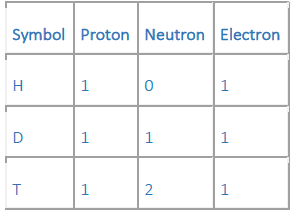Question : Write the electronic configuration of any one pair of isotopes and isobars.
Answer :  12C6 and 14C6 are isotopes, have the same electronic configuration as (2, 4)22Ne10and 22Ne11 are isobars. They have different electronic configuration as given below:
22Ne10 – 2, 8
22Ne11 – 2, 8, 1

Question : Compare the properties of electrons, protons and neutrons.Question : What are the limitations of J.J. Thomson's model of the atom?
Answer :  The limitations of J.J. Thomson's model of the atom are:
→ It could not explain the result of scattering experiment performed by rutherford.
→ It did not have any experiment support.

Question : What are the limitations of Rutherford's model of the atom?
Answer :  The limitations of Rutherford's model of the atom are
→ It failed to explain the stability of an atom.
→ It doesn't explain the spectrum of hydrogen and other atoms.

Question : Describe Bohr's model of the atom.
→ The atom consists of a small positively charged nucleus at its center.
→ The whole mass of the atom is concentrated at the nucleus and the volume of the nucleus is much smaller than the volume of the atom.
→ All the protons and neutrons of the atom are contained in the nucleus.
→ Only certain orbits known as discrete orbits of electrons are allowed inside the atom.
→ While revolving in these discrete orbits electrons do not radiate energy. These orbits or cells are represented by the letters K, L, M, N etc. or the numbers, n = 1, 2, 3, 4, . . as shown in below figure.

Question : Compare all the proposed models of an atom given in this chapter.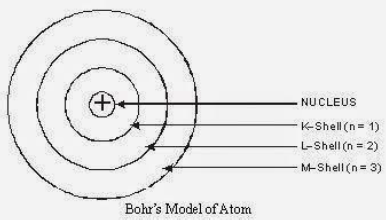Question : Compare all the proposed models of an atom given in this chapter.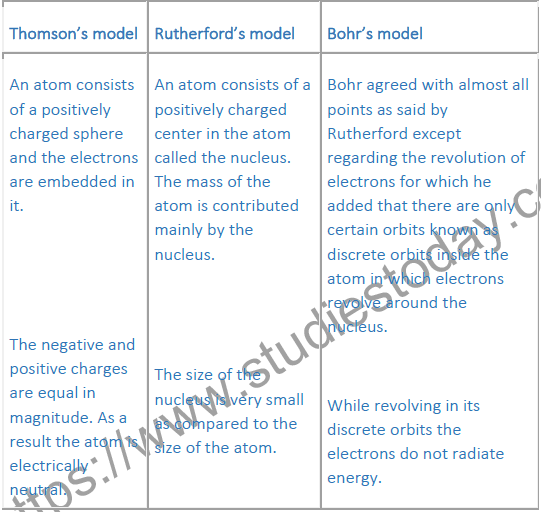Question : Summarize the rules for writing of distribution of electrons in various shells for the first eighteen elements.
Answer :  The rules for writing of the distribution of electrons in various shells for the first eighteen elements are given below.
→ If n gives the number of orbit or energy level, then 2n2 gives the maximum number of electrons possible in a given orbit or energy level.
Thus, First orbit or K-shell will have 2 electrons,
Second orbit or L-shell will have 8 electrons,
Third orbit or M-shell will have 18 electrons.
→ If it is the outermost orbit, then it should have not more than 8 electrons.
→ There should be step-wise filling of electrons in different orbits, i.e., electrons are not accompanied in a given orbit if the earlier orbits or shells are incompletely filled.

Question : Define valency by taking examples of silicon and oxygen.
Answer :  The valency of an element is the combining capacity of that element.
The valency of an element is determined by the number of valence electrons present in the atom of that element.
→ Valency of Silicon: It has electronic configuration: 2,8,4
Thus, the valency of silicon is 4 as these electrons can be shared with others to complete octet.
→ Valency of Oxygen: It has electronic configuration: 2,6
Thus, the valency of oxygen is 2 as it will gain 2 electrons to complete its octet.

Question : Explain with examples (i) Atomic number, (ii) Mass number, (iii) Isotopes and (iv) Isobars. Give any two uses of isotopes.
(i) Atomic number: The atomic number of an element is the total number of protons present in the atom of that element. For example, nitrogen has 7 protons in its atom. Thus, the atomic number of nitrogen is 7.
(ii) Mass number: The mass number of an element is the sum of the number of protons and neutrons present in the atom of that element.
For example, the atom of boron has 5 protons and 6 neutrons. So, the mass number of boron is 5 + 6 = 11.
(iii) Isotopes: These are atoms of the same element having the same atomic number, but different mass numbers. For example, chlorine has two isotopes with atomic number 17 but mass numbers 35 and 37 represented by(iv) Isobars: These are atoms having the same mass number, but different atomic numbers i.e., isobars are atoms of different elements having the same mass number. For example, Ne has atomic number 10 and sodium has atomic number 11 but both of them have mass numbers as 22 represented by -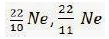Two uses of isotopes:
→ One isotope of uranium is used as a fuel in nuclear reactors.
→ One isotope of cobalt is used in the treatment of cancer.

Question : Na+ has completely filled K and L shells. Explain.
Answer :  The atomic number of sodium is 11. So, neutral sodium atom has 11 electrons and its electronic configuration is 2, 8, 1. But Na+ has 10 electrons. Out of 10, K-shell contains 2 and L-shell 8 electrons respectively. Thus, Na+ has completely filled K and L shells.

Question : If bromine atom is available in the form of, say, two isotopes 79 / 35Br (49.7%) and 81 / 35Br (50.3%), calculate the average atomic mass of bromine atom.
Answer : It is given that two isotopes of bromine are 79 / 35Br (49.7%) and 81 / 35Br (50.3%). Then, the average atomic mass of bromine atom is given by: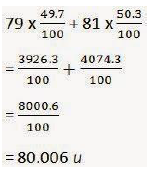Question : The average atomic mass of a sample of an element X is 16.2 u.
What are the percentages of isotopes 16 / 8 X and 18 / 8 X in the sample?
Answer :  It is given that the average atomic mass of the sample of element X is 16.2 u.
Let the percentage of isotope 18 / 8 X be y%. Thus, the percentage of isotope 16 / 8 X will be (100 - y) %.
Therefore,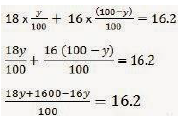18y + 1600 - 16y = 1620
2y + 1600 = 1620
2y = 1620 - 1600
y= 10
Therefore, the percentage of isotope 18 / 8 X is 10%.
And, the percentage of isotope 16 / 8 X is (100 - 10) % = 90%.

Question : If Z = 3, what would be the valency of the element? Also, name the element.
Answer : By Z = 3, we mean that the atomic number of the element is 3. Its electronic configuration is 2, 1. Hence, the valency of the element is 1 (since the outermost shell has only one electron).
Therefore, the element with Z = 3 is lithium.

Question : Composition of the nuclei of two atomic species X and Y are given as under
X Y
Protons =   6 6
Neutrons = 6 8
Give the mass numbers of X and Y. What is the relation between the two species?
Mass number of X = Number of protons + Number of neutrons = 6 + 6 = 12
Mass number of Y = Number of protons + Number of neutrons = 6 + 8 = 14
These two atomic species X and Y have the same atomic number, but different mass numbers. Hence, they are isotopes.

Question : Complete the following table.Question : Write the electronic configuration of chlorine.

Question : What determines the chemical nature of an element?

Question : What do you mean by atomic number of an element?

Question : Define cathode rays.

Question : Which elements have tendency to gain electrons?

Question : What is the relation between mass number and atomic number?

Question : Name the radioisotopes used to –
(a) determine the activity of thyroid gland.
(b) detect blood cells.

Question : What do you understand by isobars?

Question : What is the general name of the elements having 2 or 8 electrons in the valence shell of their atoms?

Question : Explain the two types of valency.

Question : Name three subatomic particles present in an atom.

Question : Who discovered neutron?

Question : Name the scientist who proposed that the atoms are indivisible?

Question : Name the scientist who presented the model of the structure of an atom for the first time.

Exam Questions NCERT Class 9 Science Chapter 4 Structure of the Atom

Question. Give the number of electron, proton and neutron in 59CO27 and 108Ag47.
Ans :
(i) Number of protons in Co = 27
(ii) Number of electrons in Co = 27
(iii) Number of neutrons in Co = 59 – 27 = 32
(iv) Number of protons in Ag = 47
(v) Number of electrons in Ag = 47
(vi) Number of neutrons in Ag = 108 – 47 = 61

Question. Do isotopes of an element have similar chemical properties?
Ans : Isotopes of an element have similar chemical properties because they have the same atomic number and valence electrons.

Question. Are noble gases inert?
Ans : The outermost shell of the atoms of noble gases are completely filled. So, they do not show affinity towards chemical reactions.

Question. What are valence electrons?
Ans : The electrons present in the outermost shell of an atom are known as valence electrons.

Question. Give two uses of isotopes in the field of medicines.
Ans : (i) An isotope of cobalt is used in the treatment of cancer.
(ii) An isotope of iodine is used in the treatment of goitre.

Question. What is meant by electronic configuration of elements?
Ans : The systematic distribution of electrons in various orbits of an atom is called electronic configuration of elements.

Question. Define atomic number. How it is denoted?
Ans : Number of protons of an atom determines its atomic number. It is denoted by ‘Z’.

Question. Why helium have zero valency?
Ans : Helium have zero valency because its outermost orbit is completely filled.

Question. Give the derivative source of sodium.
Ans : The symbol of Na for sodium is derived from its Latin name ‘Natrium’.

Question. Give drawbacks of Rutherford’s model.
Ans : Drawbacks of Rutherford’s model :
(i) Unable to explain the source of energy required for movement of electrons.
(ii) Unable to explain as to why a moving charge does not lose energy and fall into the nucleus.
(iii) It could not explain about the emission of radiations of different frequencies by different atoms when heated.
(iv) It could not explain the stability of an atom when charged electrons are moving under the attractive force of positively charged nucleus.

Question. Helium atom has an atomic mass of 4u and two protons in its nucleus. How many neutrons it have?
Ans : Mass number = Number of protons + Number of neutrons
4 =2 + No. of neutrons Number of neutrons = 4 – 2 = 2

Question. How can an atom become stable by losing or gaining electrons?
Ans : For stability of an atom it must have either 2 or 8 electrons in the outermost orbit. So, by losing or gaining electrons it reaches to the electronic configuration of the nearest noble gas.

Question. Give important properties of cathode rays.
Ans : (i) Cathode rays travel in straight line.
(ii) Cathode rays can rotate a light wheel placed in their path.
(iii) They ionise gas through which they pass.
(iv) They are deflected by magnetic field.
(v) They can penetrate through thin metallic sheet.
(vi) Mass of cathode ray particle is too small.

Question. How does an atom become a cation?
Ans : When an atom acquires positive charge by losing one or more electrons, it is called cation.

Question. How are the canal rays different from electrons in terms of charge and mass?
Ans : Electrons are negatively charged particles, mass of which is approximately 2000 1 that of canal rays.

Question. Write any two observations which support the fact that atoms are divisible.
Ans : Discovery of electrons and protons are two observations which support the fact that atoms are divisible.

Question. If an atom contains one electron and one proton, will it carry any charge or not?
Ans : It will not carry any charge because the positive charge on the proton neutralizes the negative charge on the electron.

Question. How has atomic number improved the definition of an element?
Ans : (i) Atomic number of an element = number of proton
= number of electron
(ii) Atomic number gives the position of the element in periodic table. An element can now be defined as a substance comprising of atoms all of which have same atomic number.

Question. Can the addition of neutron to the nucleus of an atom determine the atomic mass or number?
Ans : It will increase the atomic mass of the atom.

Question. What is an anion?
Ans : When an atom gains one or more electrons, it becomes negatively charged and is known as anion.

Question. Write the distribution of electrons in carbon and sodium atoms.
Ans :
Carbon: The total number of electrons in a carbon atom is 6. The distribution of electrons in carbon atom is given by: First orbit or K-shell = 2 electrons Second orbit or L-shell = 4 electrons Or, we can write the distribution of electrons in a carbon atom as 2, 4.
Sodium: The total number of electrons in a sodium atom is 11. The distribution of electrons in sodium atom is given by:
First orbit or K-shell = 2 electrons
Second orbit or L-shell = 8 electrons
Third orbit or M-shell = 1 electron
Or, we can write distribution of electrons in a sodium atom as 2, 8, 1.

Question. If K and L shells of an atom are full, then what would be the total number of electrons in the atom?
Ans : The maximum capacity of K shell is 2 electrons and L shell can accommodate maximum 8 electrons in it. Therefore, there will be ten electrons in the atom.

VALENCY:- Noble gases have fully filled outermost shell. Due to this, they are stable and they do not react with other elements. Other elements also tend to attain stable configuration by completing the octet in their outermost orbit.
This is important to note that, the number of electrons in the outermost orbit of an element is closer to octet. An element can lose or gain electron in order to complete the octet. This tendency of losing or gaining electrons imparts valency to an element. Let us take example of hydrogen. Hydrogen can readily lose or gain an electron. So, its valency is one. Now, let us take example of Hydrochloric Acid (HCl). One atom of chlorine combines with one atom of hydrogen to form hydrochloric acid. In this case, hydrogen loses one electron and thus gets +1 charge. On the other hand, chlorine gains an electron and thus gets – 1 charge. So, valency of hydrogen and chlorine are one.
Valency can be defined as the combining capacity of an atom or ion.
Example :
Hydrogen molecule - Hydrogen has only one electron in its outermost orbit, thus it requires one more electrons to complete its outermost orbit. Therefore, in order to complete outermost orbit, hydrogen shares one electron with another hydrogen atom and form H2 (hydrogen molecule).
In the case of LiCl (Lithium chloride) - Lithium has three electrons in its outermost orbit and chlorine has seven electrons in its outermost orbit. Thus in order to make outermost orbit completely filled lithium loses one electrons and chlorine gains one electron. After losing one electron, lithium has two electrons in its outermost orbit and after gaining one electron, chlorine has eight electrons in its outermost orbit. And they form LiCl (Lithium chloride)

 CBSE Class 9 Science Matter in Our Surroundings Assignment Set A CBSE Class 9 Science Matter in Our Surroundings Assignment Set B
 CBSE Class 9 Science Gravitation Set A CBSE Class 9 Science Gravitation Set B
 CBSE Class 9 Science Work and Energy assignment
 CBSE Class 9 Science Sound Assignment
 CBSE Class 9 Science Why do we Fall ill Assignment
 CBSE Class 9 Science Natural Resources assignment
 CBSE Class 9 Science Improvement in Food Resources assignment
 CBSE Class 9 Science Is Matter around Us Pure assignment
 CBSE Class 9 Science Atoms and Molecules assignment
 CBSE Class 9 Science Structure of the Atom assignment
 CBSE Class 9 Science The Fundamental Unit of Life Assignment Set A CBSE Class 9 Science The Fundamental Unit of Life Assignment Set B
 CBSE Class 9 Science Tissues Assignment Set A CBSE Class 9 Science Tissues Assignment Set B
 CBSE Class 9 Science Diversity in Living Organisms assignment
 CBSE Class 9 Science Motion Assignment Set A CBSE Class 9 Science Motion Assignment Set B
 CBSE Class 9 Science Force and Laws of Motion Assignment Set A CBSE Class 9 Science Force and Laws of Motion Assignment Set B
 CBSE Class 9 Science Collection of Assignments CBSE Class 9 Science OTBA Clean Air Shared Concern assignment CBSE Class 9 Science OTBA Environment Development From Hills assignment CBSE Class 9 Science Physics Question bank

Tags